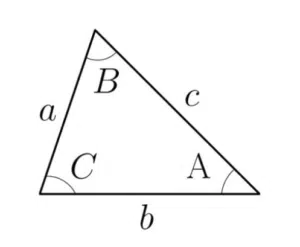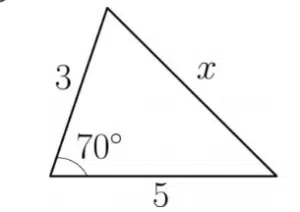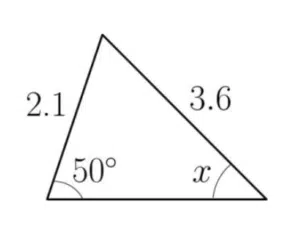# Non right angled trianglesFor right-angled triangles, we have Pythagoras’ Theorem and SOHCAHTOA. However, these methods do not work for non-right angled triangles. For non-right angled triangles, we have the cosine rule, the sine rule and a new expression for finding area.

In order to use these rules, we require a technique for labelling the sides and angles of the non-right angled triangle. This may mean that a relabelling of the features given in the actual question is needed. See the non-right angled triangle given here. Angle A is opposite side a, angle B is opposite side B and angle C is opposite side c. We determine the best choice by which formula you remember in the case of the cosine rule and what information is given in the question but you must always have the UPPER CASE angle OPPOSITE the LOWER CASE side.

## The Cosine Rule

These formulae represent the cosine rule. Note that it is not necessary to memorise all of them – one will suffice, since a relabelling of the angles and sides will give you the others. Students tend to memorise the bottom one as it is the one that looks most like Pythagoras.

We use the cosine rule to find a missing side when all sides and an angle are involved in the question. It may also be used to find a missing angle if all the sides of a non-right angled triangle are known. See Examples 1 and 2.

The Cosine Rule

$a^2=b^2+c^2-2bc\cos(A)$
$b^2=a^2+c^2-2ac\cos(B)$
$c^2=a^2+b^2-2ab\cos(C)$

## The Sine Rule

This formula represents the sine rule. The sine rule can be used to find a missing angle or a missing side when two corresponding pairs of angles and sides are involved in the question. This is different to the cosine rule since two angles are involved. This is a good indicator to use the sine rule in a question rather than the cosine rule. See Example 3.

Note that when using the sine rule, it is sometimes possible to get two answers for a given angle\side length, both of which are valid. See Example 4.

The Sine Rule

$\frac{a}{\sin(A)}=\frac{b}{\sin(B)}=\frac{c}{\sin(C)}$

or

$\frac{\sin(A)}{a}=\frac{\sin(B)}{b}=\frac{\sin(C)}{c}$

## The Area of a Non-Right Angled Triangle

These formulae represent the area of a non-right angled triangle. Again, it is not necessary to memorise them all – one will suffice (see Example 2 for relabelling). It is the analogue of a half base times height for non-right angled triangles.

Note that to maintain accuracy, store values on your calculator and leave rounding until the end of the question. You can round when jotting down working but you should retain accuracy throughout calculations. See Examples 5 and 6.

The Area of a Non-Right Angled Triangle

$\frac{1}{2}ab\sin(C)$

$\frac{1}{2}bc\sin(A)$

$\frac{1}{2}ac\sin(B)$

## Examples of Non Right Angled Triangles

Find the length of the side marked x in the following triangle:The triangle PQR has sides $PQ=6.5$cm, $QR=9.7$cm and $PR = c$cm. Angle $QPR$ is $122^\circ$. Find the value of $c$.

Find the angle marked $x$ in the following triangle to 3 decimal places:In triangle $XYZ$, length $XY=6.14$m, length $YZ=3.8$m and the angle at $X$ is $27^\circ$. Sketch the two possibilities for this triangle and find the two possible values of the angle at $Y$ to 2 decimal places.

Find the area of this triangle.

Find the area of the triangle with sides 22km, 36km and 47km to 1 decimal place.